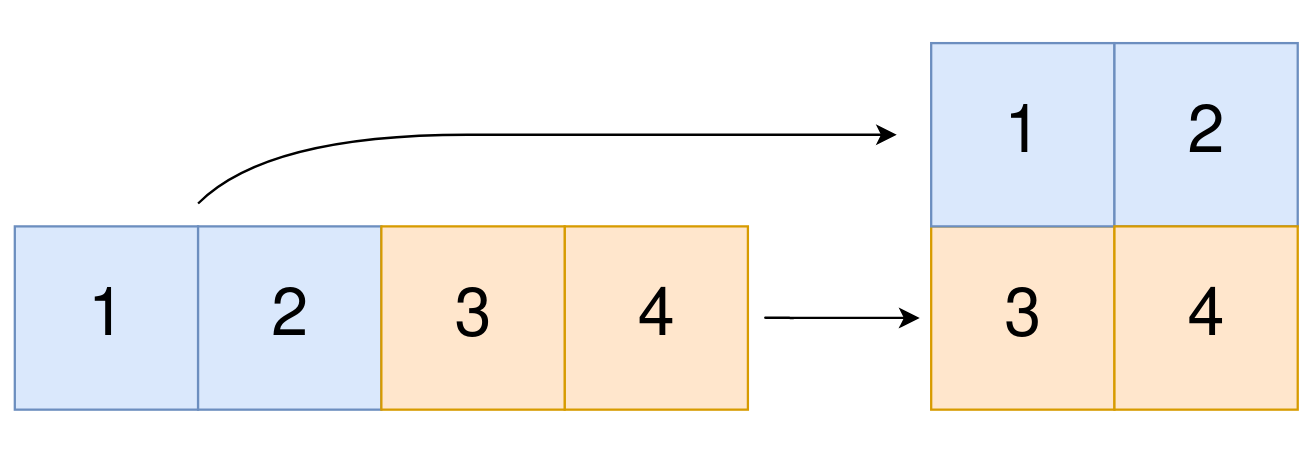# Convert 1D Array into 2D Array

Easy
6
59.3% Acceptance

In this lab, you will be tasked with converting a given 0-indexed 1-dimensional (1D) integer array `original` into a 2-dimensional (2D) array. You will be given two integers, `m` and `n`, representing the desired number of rows and columns, respectively, for the resulting 2D array. Your goal is to create a 2D array with `m` rows and `n` columns using all the elements from `original`.

The elements from indices `0` to `n - 1` (inclusive) of `original` should form the first row of the constructed 2D array, the elements from indices `n` to `2 * n - 1` (inclusive) should form the second row of the constructed 2D array, and so on.

Your function `construct2DArray` should return an `m x n` 2D array constructed according to the above procedure, or an empty 2D array if it is impossible.

## Examples

Example 1:Input: original = [1,2,3,4], m = 2, n = 2 Output: [[1,2],[3,4]] Explanation: The constructed 2D array should contain 2 rows and 2 columns. The first group of n = 2 elements in original, [1,2], becomes the first row in the constructed 2D array. The second group of n = 2 elements in original, [3,4], becomes the second row in the constructed 2D array.

Example 2:

Input: original = [1,2,3], m = 1, n = 3 Output: [[1,2,3]] Explanation: The constructed 2D array should contain 1 row and 3 columns. Put all three elements in the original into the first row of the constructed 2D array.

Example 3:

Input: original = [1,2], m = 1, n = 1 Output: [] Explanation: There are 2 elements in the original. It is impossible to fit 2 elements in a 1x1 2D array, so return an empty 2D array.

## Constraints

• `1 <= original.length <= 5 * 104`
• `1 <= original[i] <= 105`
• `1 <= m, n <= 4 * 104`

Remember to export the `construct2DArray` function so that it can be imported and tested in the evaluation script.

``````export function construct2DArray(original, m, n) {